# 一文弄懂计数排序算法！

### 03 基础版代码实现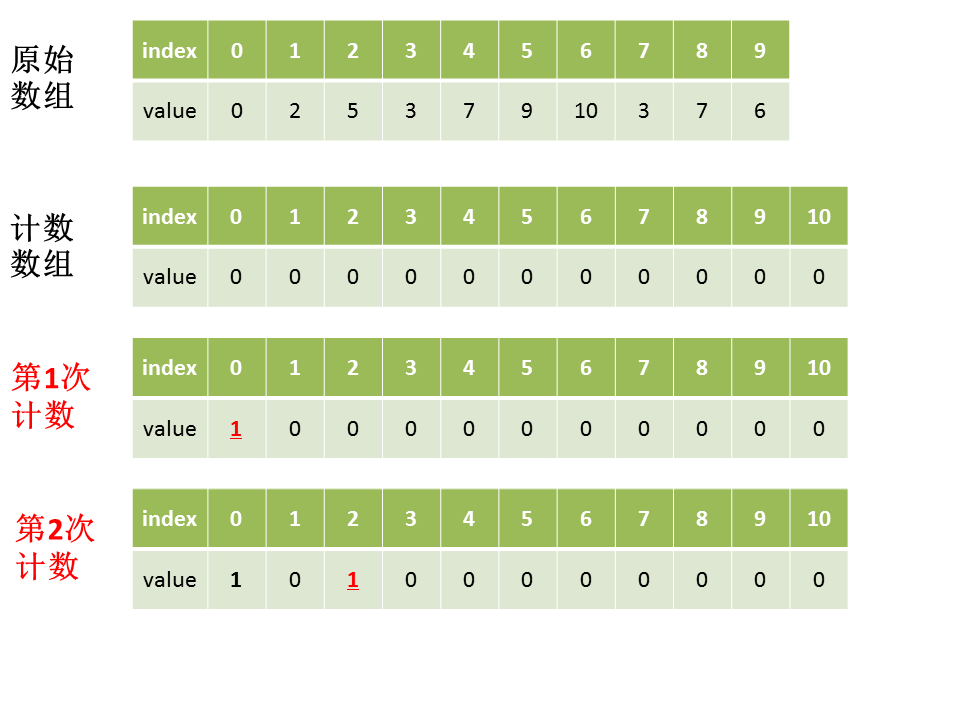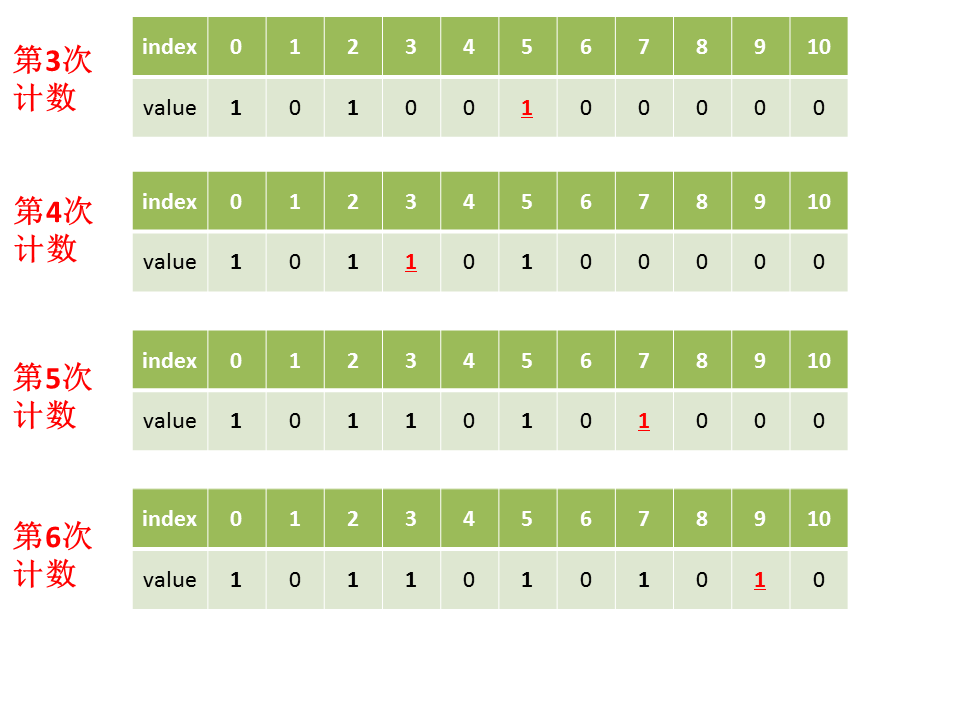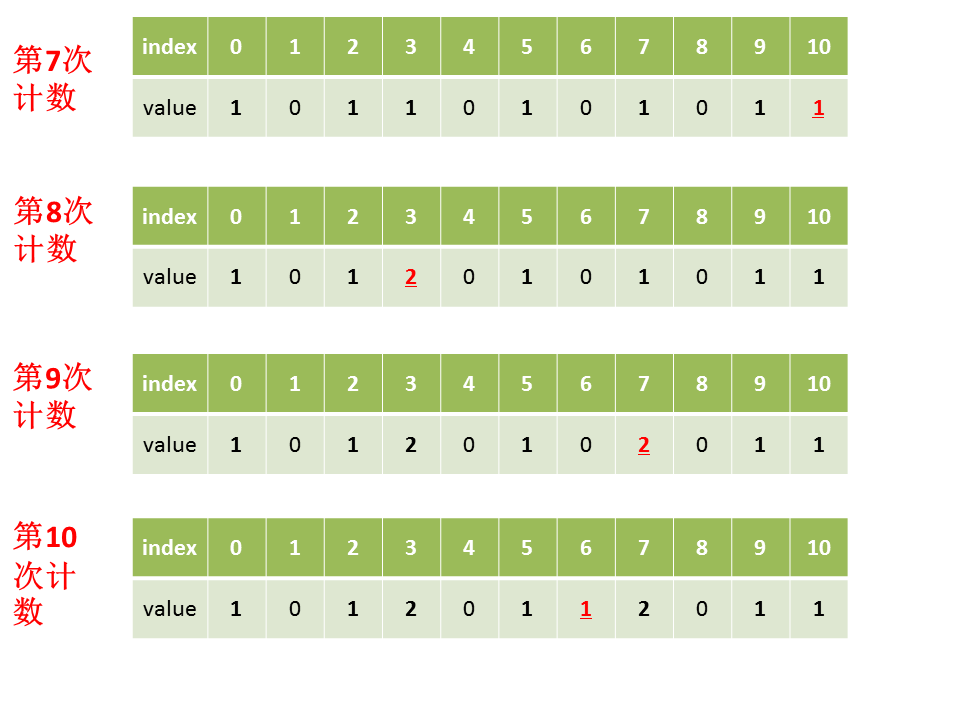public int[] countSort(int[] A) {
// 找出数组A中的最大值
int max = Integer.MIN_VALUE;
for (int num : A) {
max = Math.max(max, num);
}
// 初始化计数数组count
int[] count = new int[max+1];
// 对计数数组各元素赋值
for (int num : A) {
count[num]++;
}
// 创建结果数组
int[] result = new int[A.length];
// 创建结果数组的起始索引
int index = 0;
// 遍历计数数组，将计数数组的索引填充到结果数组中
for (int i=0; i<count.length; i++) {
while (count[i]>0) {
result[index++] = i;
count[i]--;
}
}
// 返回结果数组
return result;
}


### 04 优化版

public int[] countSort2(int[] A) {
// 找出数组A中的最大值、最小值
int max = Integer.MIN_VALUE;
int min = Integer.MAX_VALUE;
for (int num : A) {
max = Math.max(max, num);
min = Math.min(min, num);
}
// 初始化计数数组count
// 长度为最大值减最小值加1
int[] count = new int[max-min+1];
// 对计数数组各元素赋值
for (int num : A) {
// A中的元素要减去最小值，再作为新索引
count[num-min]++;
}
// 创建结果数组
int[] result = new int[A.length];
// 创建结果数组的起始索引
int index = 0;
// 遍历计数数组，将计数数组的索引填充到结果数组中
for (int i=0; i<count.length; i++) {
while (count[i]>0) {
// 再将减去的最小值补上
result[index++] = i+min;
count[i]--;
}
}
// 返回结果数组
return result;
}


### 05 进阶版步骤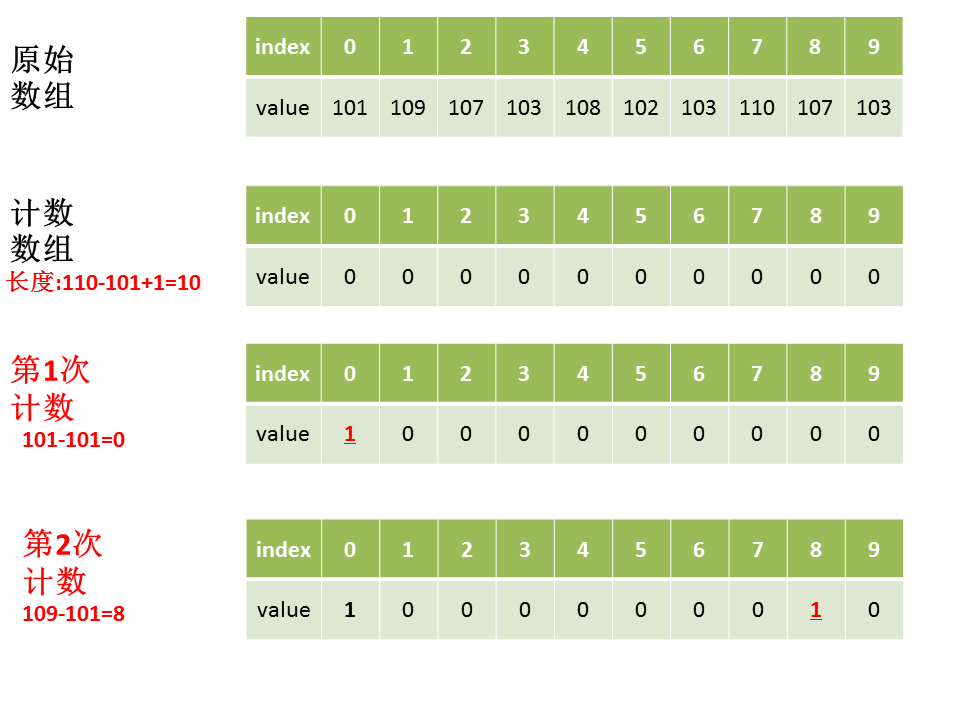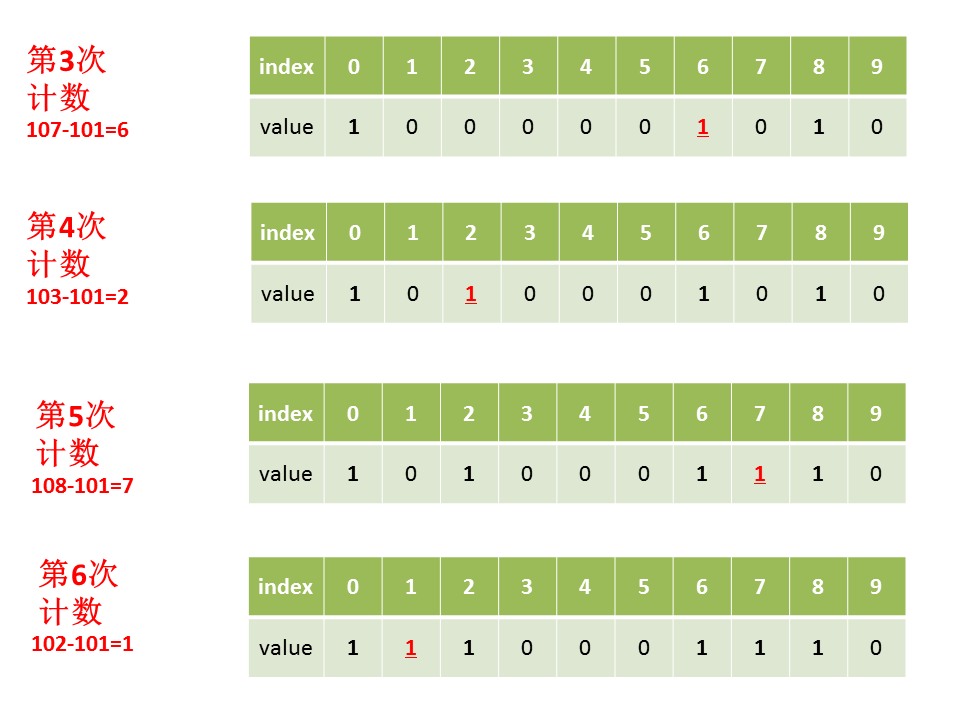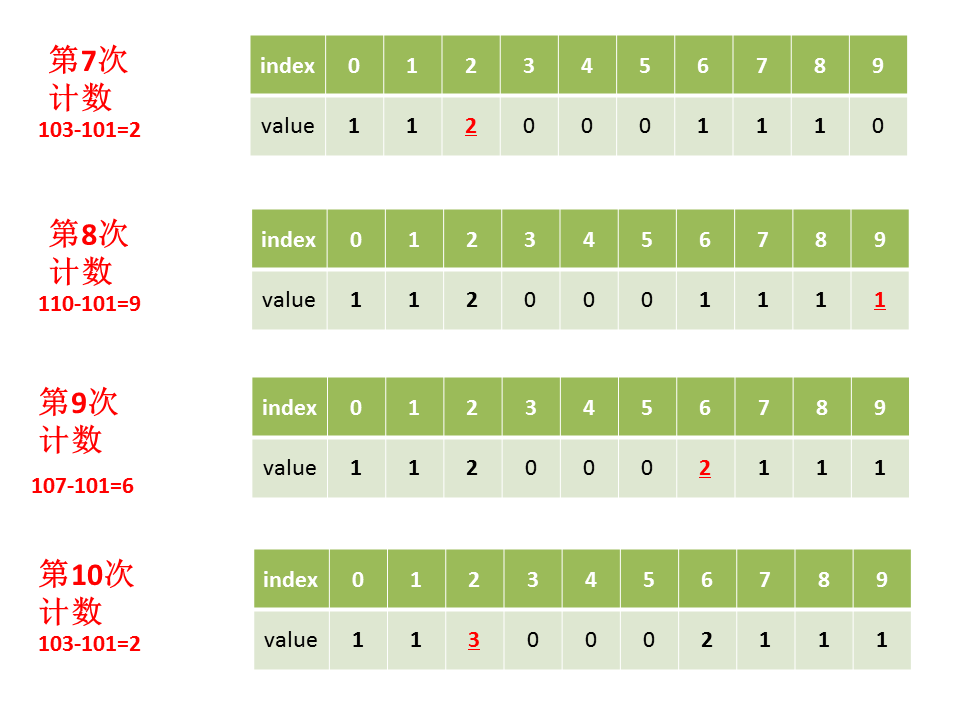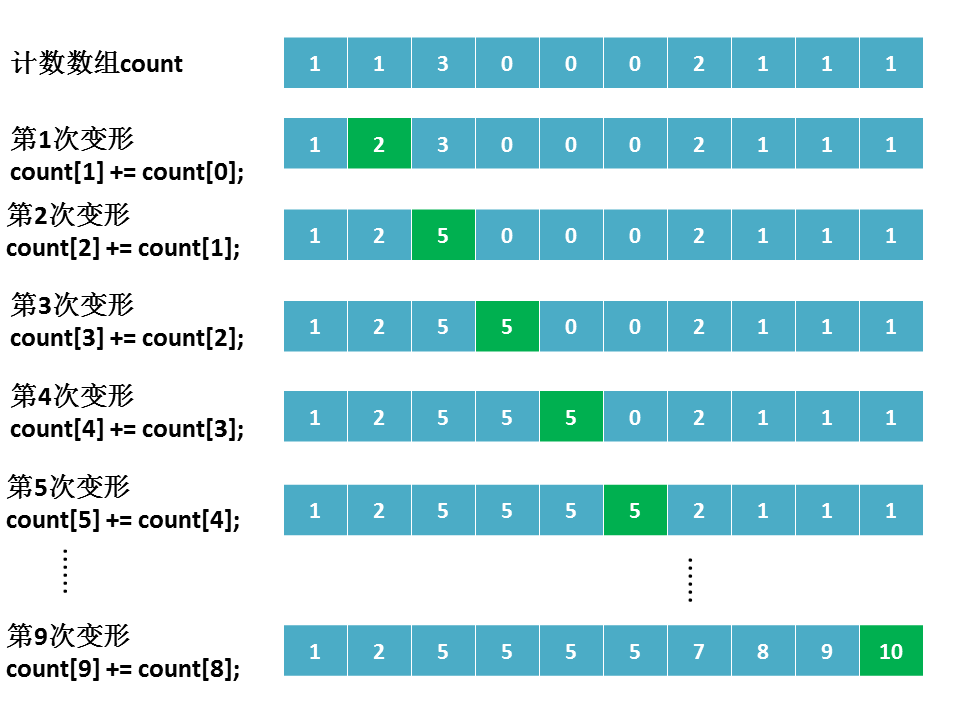### 06 进阶版代码实现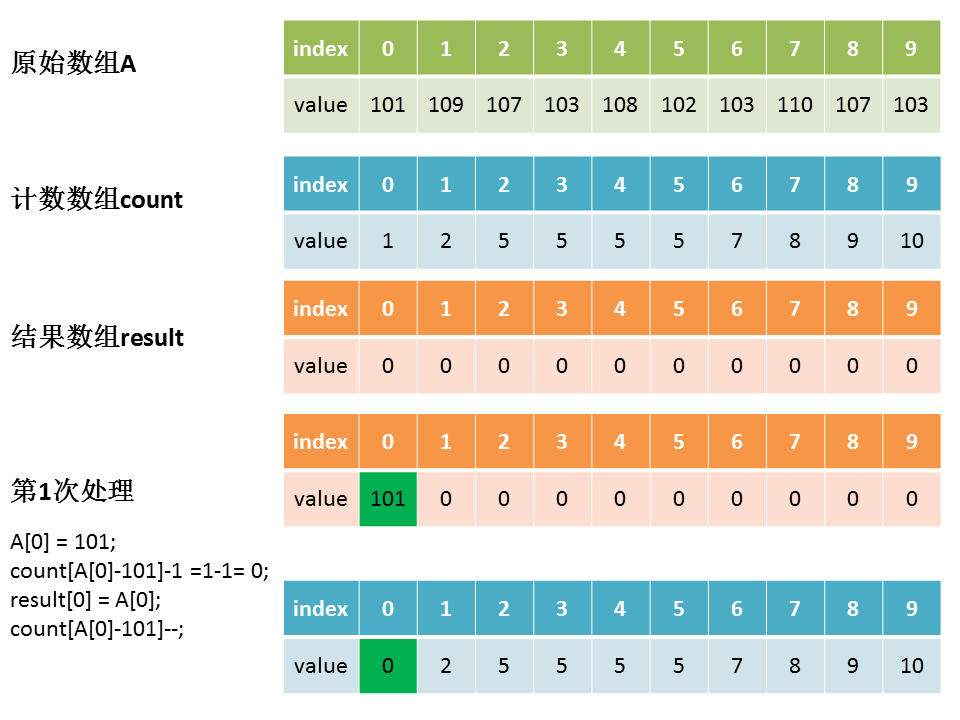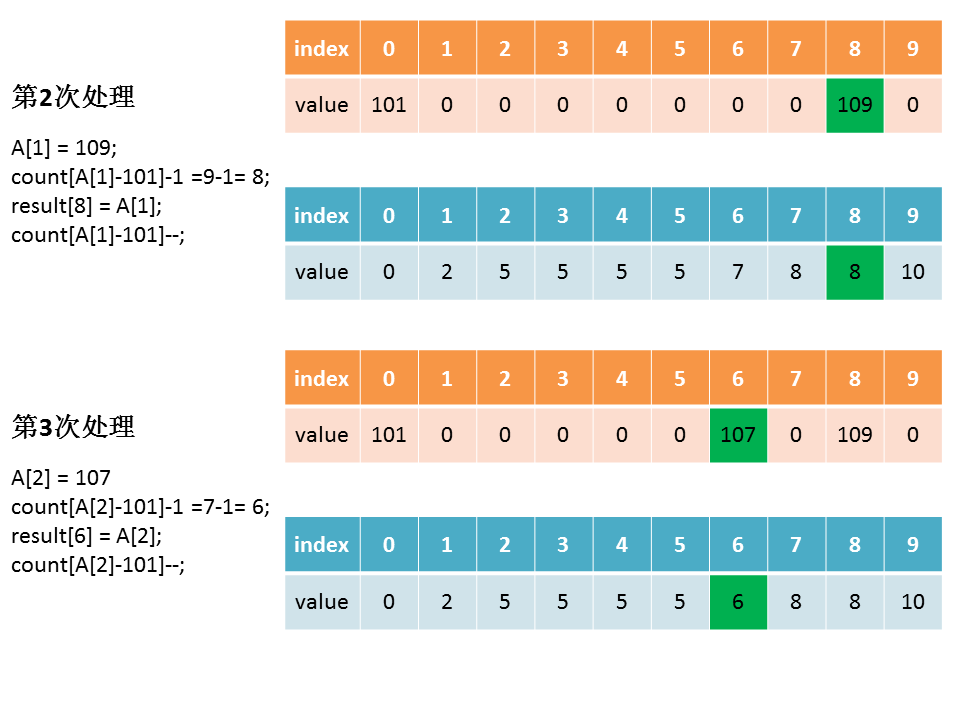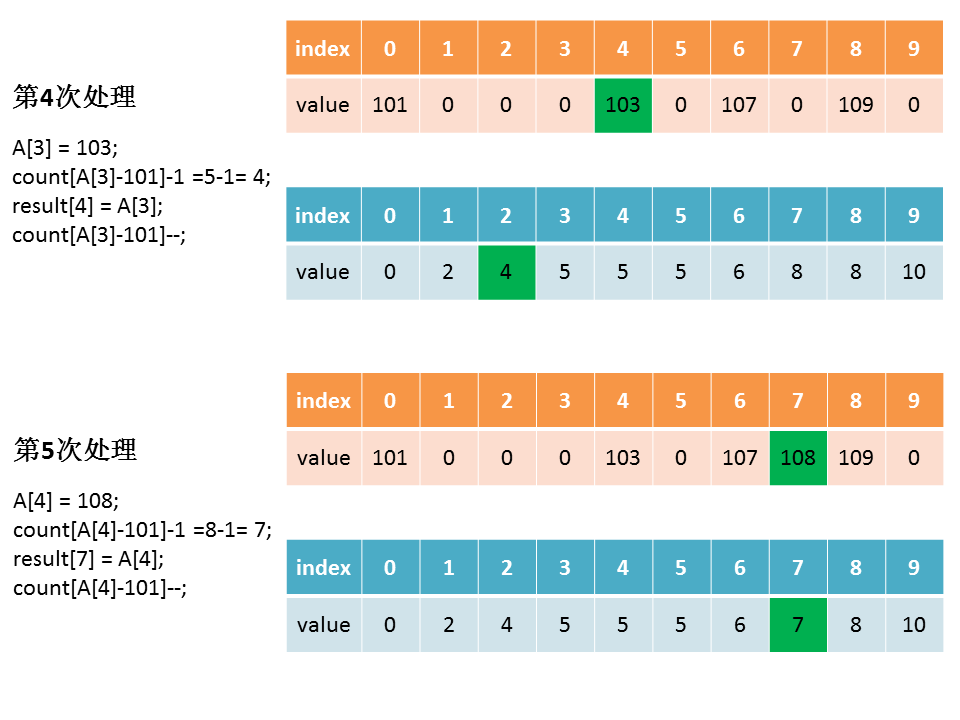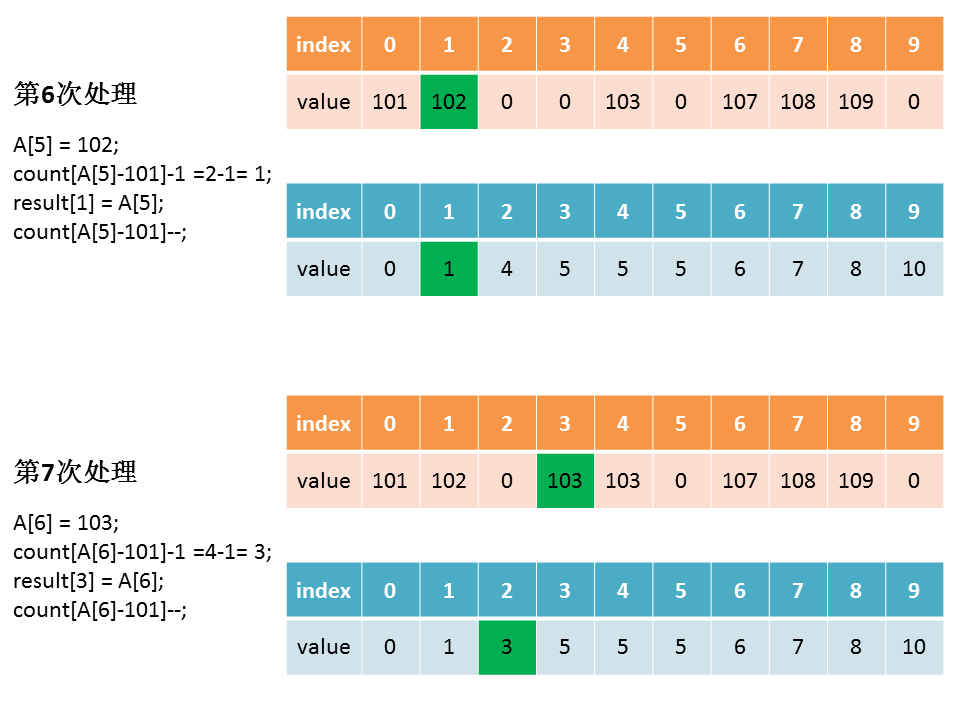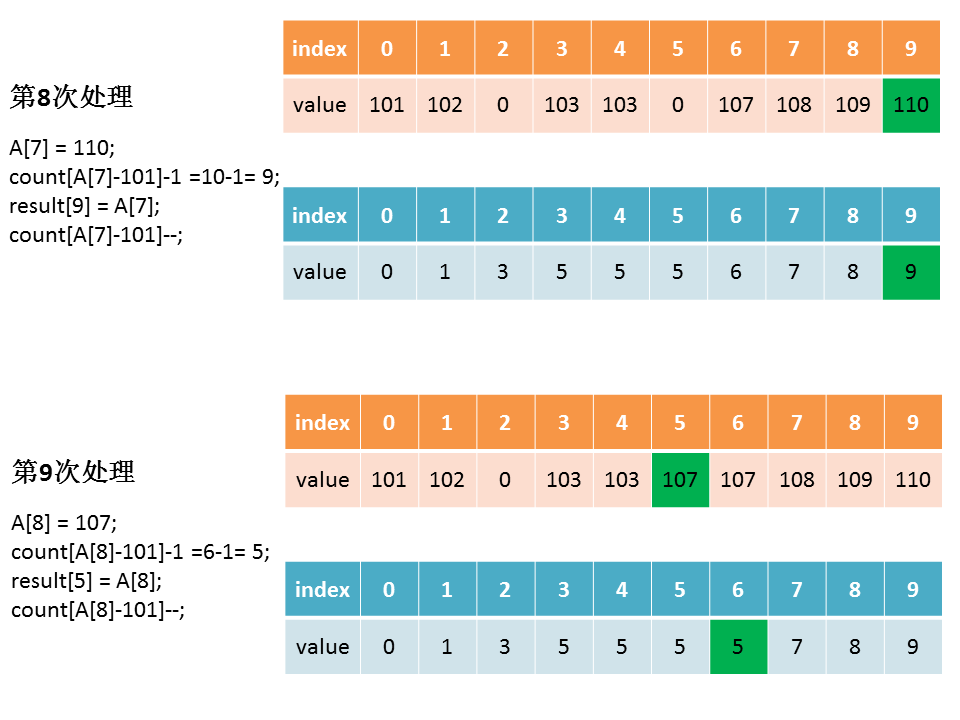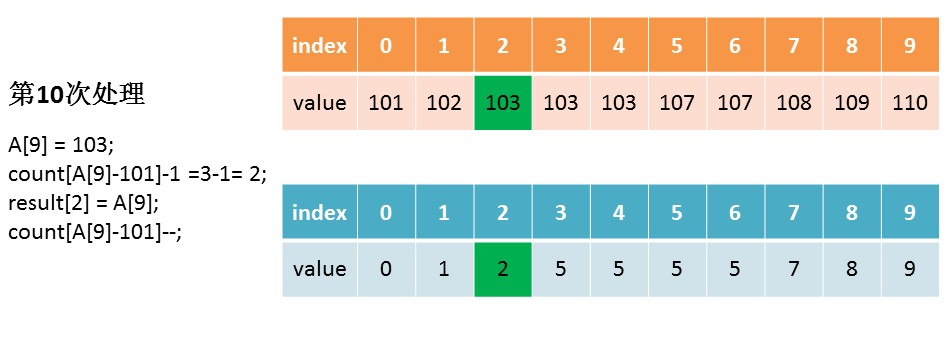public int[] countSort3(int[] A) {
// 找出数组A中的最大值、最小值
int max = Integer.MIN_VALUE;
int min = Integer.MAX_VALUE;
for (int num : A) {
max = Math.max(max, num);
min = Math.min(min, num);
}
// 初始化计数数组count
// 长度为最大值减最小值加1
int[] count = new int[max-min+1];
// 对计数数组各元素赋值
for (int num : A) {
// A中的元素要减去最小值，再作为新索引
count[num-min]++;
}
// 计数数组变形，新元素的值是前面元素累加之和的值
for (int i=1; i<count.length; i++) {
count[i] += count[i-1];
}
// 创建结果数组
int[] result = new int[A.length];
// 遍历A中的元素，填充到结果数组中去
for (int j=0; j<A.length; j++) {
result[count[A[j]-min]-1] = A[j];
count[A[j]-min]--;
}
return result;
}


### 07 进阶版的延伸之一

public int[] countSort4(int[] A) {
// 找出数组A中的最大值、最小值
int max = Integer.MIN_VALUE;
int min = Integer.MAX_VALUE;
for (int num : A) {
max = Math.max(max, num);
min = Math.min(min, num);
}
// 初始化计数数组count
// 长度为最大值减最小值加1
int[] count = new int[max-min+1];
// 对计数数组各元素赋值
for (int num : A) {
// A中的元素要减去最小值，再作为新索引
count[num-min]++;
}
// 计数数组变形，新元素的值是前面元素累加之和的值
for (int i=1; i<count.length; i++) {
count[i] += count[i-1];
}
// 创建结果数组
int[] result = new int[A.length];
// 遍历A中的元素，填充到结果数组中去，从后往前遍历
for (int j=A.length-1; j>=0; j--) {
result[count[A[j]-min]-1] = A[j];
count[A[j]-min]--;
}
return result;
}


### 08 进阶版的延伸之二

public int[] countSort5(int[] A) {
// 找出数组A中的最大值、最小值
int max = Integer.MIN_VALUE;
int min = Integer.MAX_VALUE;
for (int num : A) {
max = Math.max(max, num);
min = Math.min(min, num);
}
// 初始化计数数组count
// 长度为最大值减最小值加1，再加1
int[] count = new int[(max-min+1)+1];
// 对计数数组各元素赋值，count永远为0
for (int num : A) {
// A中的元素要减去最小值再加上1，再作为新索引
count[num-min+1]++;
}
// 计数数组变形，新元素的值是前面元素累加之和的值
for (int i=1; i<count.length; i++) {
count[i] += count[i-1];
}
// 创建结果数组
int[] result = new int[A.length];
// 遍历A中的元素，填充到结果数组中去，从前往后遍历
for (int j=0; j<A.length; j++) {
// 如果后面遇到相同的元素，在前面元素的基础上往后排
// 如此就保证了原始数组中相同元素的原始排序
result[count[A[j]-min]] = A[j];
count[A[j]-min]++;
}
return result;
}


### 09 小结

posted @ 2019-07-17 08:41  程序员小川  阅读(31543)  评论(8编辑  收藏  举报